Skip to content
Related Articles
Facebook API | Set-3
• Last Updated : 25 Jun, 2019

Prerequisite: Facebook API | Set-1, Set-2

In this article we will be discussing three methods:

1. search
2. get_connections
3. get_allconnections

## `search `method:

The valid value types are place and placetopic.

Parameters:
id: It is a string containing a valid value.
args: This is optional and they need to passed a query params.

Example: Get details of all places that are near Connaught Place Delhi. The Latitude and Longitude of Connaught Place are – 28.6304, 77.2177

 `import` `json``import` `facebook`` ` `def` `main():``    ``token ``=` `"Please replace with your access token"``    ``graph ``=` `facebook.GraphAPI(token)`` ` `    ``places ``=` `graph.search(``type` `=``'place'``, center ``=``'28.6304, 77.2177'``, ``                                            ``fields ``=``'name, location'``)`` ` `    ``for` `place ``in` `places[``'data'``]:``        ``print``(``'%s %s'` `%``(place[``'name'``].encode(), place[``'location'``].get(``'zip'``)))``     ` `if` `__name__ ``=``=` `'__main__'``:``    ``main()`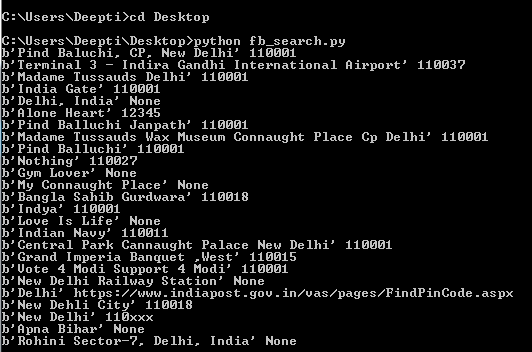Please refer to the link Search Reference for complete list of fields that can used.

## `get_connections `method :

This methods aims at returning all connections or we can say Edges for the mentioned object as dict.

Parameters:
id:A string specifying the unique id for the resource under question.
connection name: A string specifying the connection or edges between the objects.

If connection name parameter is left empty then `get_connections `method will simply return the basic information of the authenticated user.

Example #1: We want to find out the total count of the number of Friends of the active user.

 `import` `json``import` `facebook`` ` `def` `main():``    ``token ``=` `"Please replace this with your access token"``    ``graph ``=` `facebook.GraphAPI(token)``    ``friends ``=` `graph.get_connections(``id` `=``'me'``, connection_name ``=``'friends'``)``    ``print``(json.dumps(friends, indent ``=` `4``))`` ` `if` `__name__ ``=``=` `'__main__'``:``    ``main()`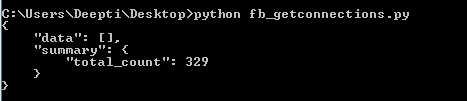Example #2: We want to get list of all posts from a page. For this, we will be using get_connections and later on, we will demonstrate how to use `get_all_connections `method.

 `import` `json``import` `facebook`` ` `def` `main():``    ``token ``=` `"Please replace this with your PAGE Access Token"``    ``graph ``=` `facebook.GraphAPI(token)``    ``posts_25 ``=` `graph.get_connections(``id` `=``'PAGE_ID'``, connection_name ``=``'posts'``,``                                                  ``fields ``=``'id, created_time'``)`` ` `    ``print``(json.dumps(posts_25, indent ``=` `4``))`` ` `if` `__name__ ``=``=` `'__main__'``:``    ``main() `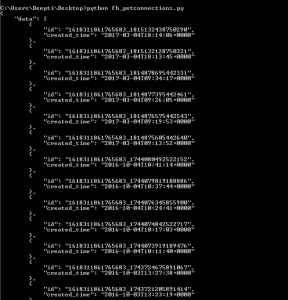Note: This example by default print latest 25 posts. You can add a filter for the post using limit(…) to set the limit for the number of the posts.

Example #3: In this example we will use get_connections method to print all the comments for the posts in `reverse_chronological `order, show sub comments including hidden comments and also show the total count of the comments.

 `import` `json``import` `facebook`` ` `def` `main():``    ``token ``=` `"Please replace this with your PAGE Access Token"``    ``graph ``=` `facebook.GraphAPI(token)``    ``posts_25 ``=` `graph.get_connections(``id` `=``'POST_ID'``, connection_name ``=``'comments'``, ``                                     ``include_hidden ``=` `True``, order ``=``'reverse_chronological'``,``                                     ``filter` `=``'stream'``, summary ``=``'total_count'``)`` ` `    ``print``(json.dumps(posts_25, indent ``=` `4``))`` ` `if` `__name__ ``=``=` `'__main__'``:``    ``main() `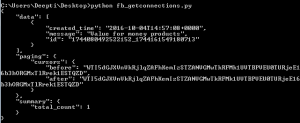## `get_all_connections `method :

Iterates over all pages returned by a get_connections call and yields the individual items.

Parameters:
id:A string specifying the unique id for the resource under question.
connection name: A string specifying the connection or edges between the objects.

Example #1: In this example I have used get_all_connections to list all the posts from a Page listed using the since datetime parameter.

 `import` `json``import` `facebook``from` `datetime ``import` `datetime``  ` `def` `main():``    ``token ``=` `"Please replace this with your PAGE Access Token"``    ``graph ``=` `facebook.GraphAPI(token)``    ``posts_all ``=` `graph.get_all_connections(``id` `=``'PAGE_ID'``, connection_name ``=``'posts'``,``                                           ``fields ``=``'created_time, id'``,``                                           ``since ``=` `datetime(``2017``, ``1``, ``1``, ``0``, ``0``, ``0``))`` ` `    ``for` `ind, post ``in` `enumerate``(posts_all):``     ``print``(json.dumps(ind, indent ``=` `4``))``     ``print``(json.dumps(post, indent ``=` `4``))``     ` `  ` `if` `__name__ ``=``=` `'__main__'``:``    ``main()`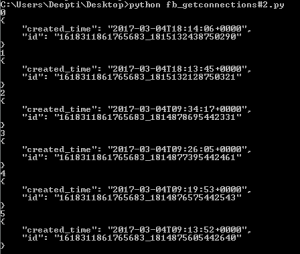References:

1. https://developers.facebook.com/docs/marketing-api/insights/parameters
2. https://developers.facebook.com/docs/graph-api/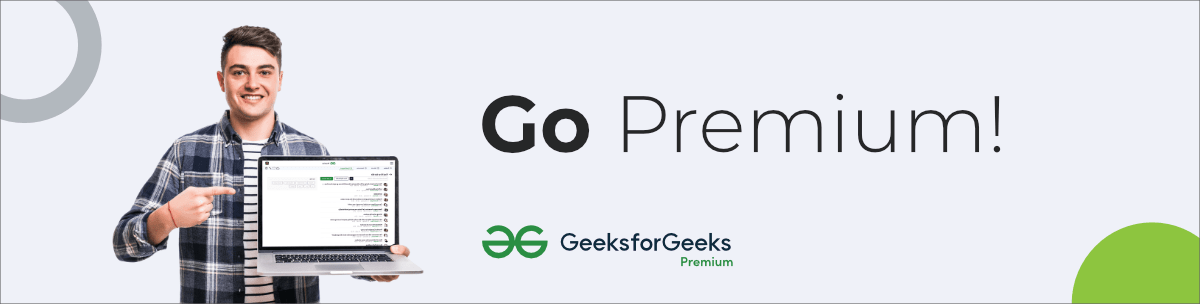My Personal Notes arrow_drop_up